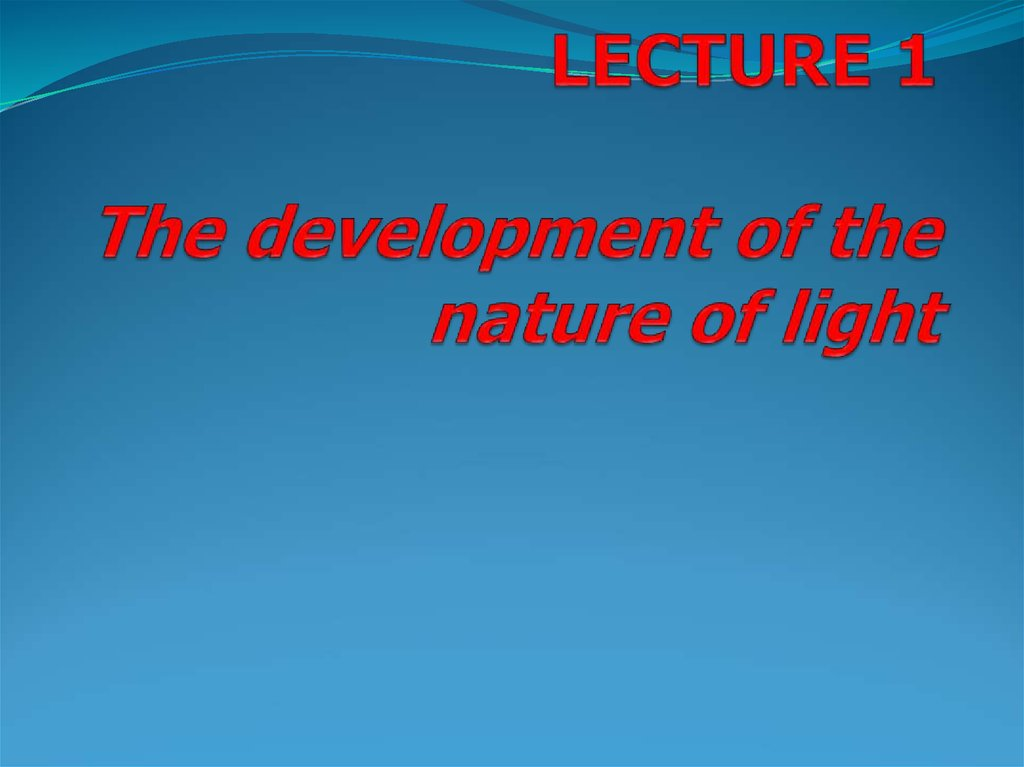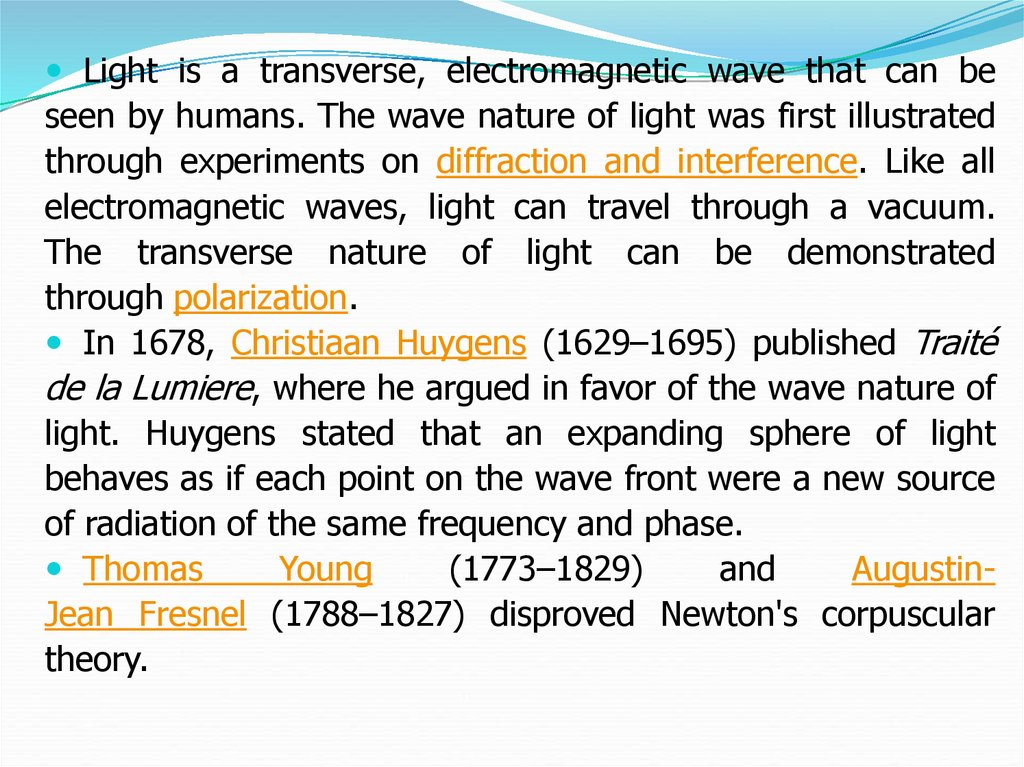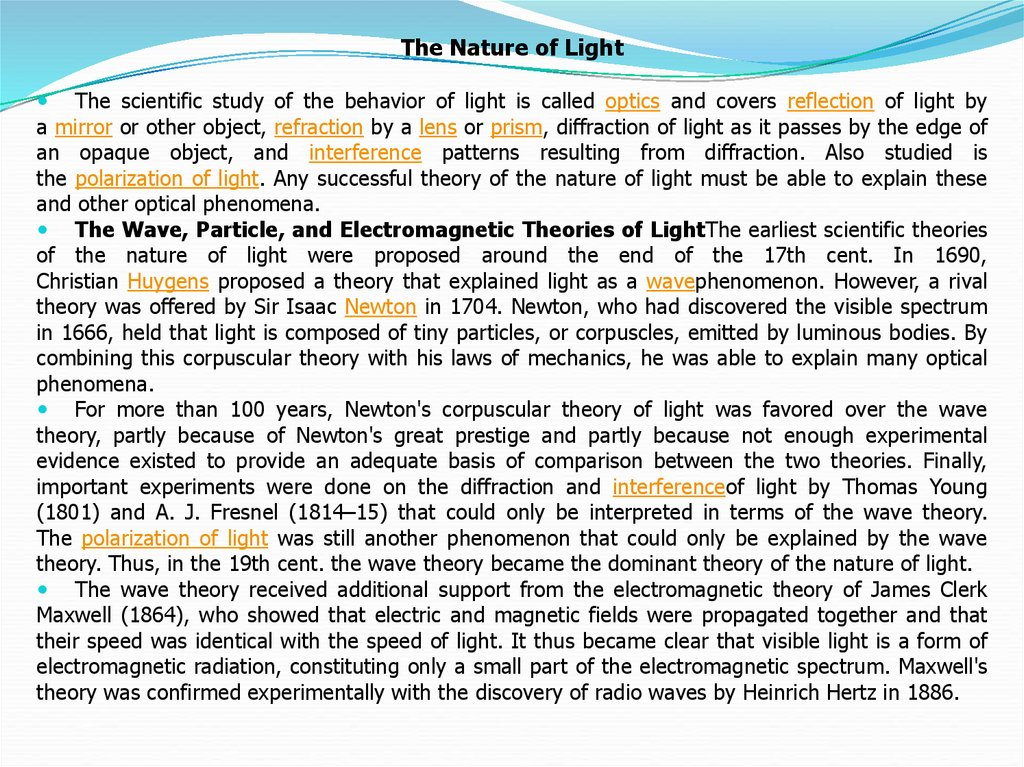# The development of the nature of light

## 2.

Light is a transverse, electromagnetic wave that can be
seen by humans. The wave nature of light was first illustrated
through experiments on diffraction and interference. Like all
electromagnetic waves, light can travel through a vacuum.
The transverse nature of light can be demonstrated
through polarization.
In 1678, Christiaan Huygens (1629–1695) published Traité
de la Lumiere, where he argued in favor of the wave nature of
light. Huygens stated that an expanding sphere of light
behaves as if each point on the wave front were a new source
of radiation of the same frequency and phase.
Thomas
Young
(1773–1829)
and
AugustinJean Fresnel (1788–1827) disproved Newton's corpuscular
theory.

## 3.

The Nature of Light
The scientific study of the behavior of light is called optics and covers reflection of light by
a mirror or other object, refraction by a lens or prism, diffraction of light as it passes by the edge of
an opaque object, and interference patterns resulting from diffraction. Also studied is
the polarization of light. Any successful theory of the nature of light must be able to explain these
and other optical phenomena.
The Wave, Particle, and Electromagnetic Theories of LightThe earliest scientific theories
of the nature of light were proposed around the end of the 17th cent. In 1690,
Christian Huygens proposed a theory that explained light as a wavephenomenon. However, a rival
theory was offered by Sir Isaac Newton in 1704. Newton, who had discovered the visible spectrum
in 1666, held that light is composed of tiny particles, or corpuscles, emitted by luminous bodies. By
combining this corpuscular theory with his laws of mechanics, he was able to explain many optical
phenomena.
For more than 100 years, Newton's corpuscular theory of light was favored over the wave
theory, partly because of Newton's great prestige and partly because not enough experimental
evidence existed to provide an adequate basis of comparison between the two theories. Finally,
important experiments were done on the diffraction and interferenceof light by Thomas Young
(1801) and A. J. Fresnel (1814–15) that could only be interpreted in terms of the wave theory.
The polarization of light was still another phenomenon that could only be explained by the wave
theory. Thus, in the 19th cent. the wave theory became the dominant theory of the nature of light.
The wave theory received additional support from the electromagnetic theory of James Clerk
Maxwell (1864), who showed that electric and magnetic fields were propagated together and that
their speed was identical with the speed of light. It thus became clear that visible light is a form of
electromagnetic radiation, constituting only a small part of the electromagnetic spectrum. Maxwell's
theory was confirmed experimentally with the discovery of radio waves by Heinrich Hertz in 1886.

## 4.

Modern Theory of the Nature of Light
With the acceptance of the electromagnetic theory of light, only two general problems
remained. One of these was that of the luminiferous ether, a hypothetical medium suggested
as the carrier of light waves, just as air or water carries sound waves. The ether was assumed
to have some very unusual properties, e.g., being massless but having high elasticity. A
number of experiments performed to give evidence of the ether, most notably by A. A.
Michelson in 1881 and by Michelson and E. W. Morley in 1887, failed to support the ether
hypothesis. With the publication of the special theory of relativity in 1905 by Albert Einstein,
the ether was shown to be unnecessary to the electromagnetic theory.
The second main problem, and the more serious of the two, was the explanation of
various phenomena, such as the photoelectric effect, that involved the interaction of light with
matter. Again the solution to the problem was proposed by Einstein, also in 1905. Einstein
extended the quantum theory of thermal radiation proposed by Max Planck in 1900 to cover
not only vibrations of the source of radiation but also vibrations of the radiation itself. He thus
suggested that light, and other forms of electromagnetic radiation as well, travel as tiny
bundles of energy called light quanta, or photons. The energy of each photon is directly
proportional to its frequency.
With the development of the quantum theory of atomic and molecular structure by Niels
Bohr and others, it became apparent that light and other forms of electromagnetic radiation
are emitted and absorbed in connection with energy transitions of the particles of the
substance radiating or absorbing the light. In these processes, the quantum, or particle, nature
of light is more important than its wave nature. When the transmission of light is under
consideration, however, the wave nature dominates over the particle nature. In 1924, Louis de
Broglie showed that an analogous picture holds for particle behavior, with moving particles
having certain wavelike properties that govern their motion, so that there exists a
complementarity principle). The quantum theory of light has successfully explained all aspects
of the behavior of light.

## 5.

Refraction of Light
Refraction is the bending of a wave when it enters a medium where its speed is
different. The refraction of light when it passes from a fast medium to a slow medium
bends the light ray toward the normal to the boundary between the two media. The
amount of bending depends on the indices of refraction of the two media and is
described quantitatively bySnell's Law.
As the speed of light is reduced in the slower medium, the wavelength is shortened
proportionately. The frequency is unchanged; it is a characteristic of the source of the
light and unaffected by medium changes.
Refraction is responsible for image
formation by lenses and the eye.

## 6.

Refraction is the change in direction of propagation of a wave due
to a change in its transmission medium.
The phenomenon is explained by the conservation of
energy and conservation of momentum. Due to change of medium,
the phase velocity of the wave is changed but its frequency remains
constant. This is most commonly observed when a wave passes from
onemedium to another at any angle other than 0° from the normal.
Refraction of light is the most commonly observed phenomenon, but
any type of wave can refract when it interacts with a medium, for
example when sound waves pass from one medium into another or
when water waves move into water of a different depth. Refraction is
described by Snell's law, which states that for a given pair of media
and a wave with a single frequency, the ratio of the sines of the angle
of incidence θ1 and angle of refraction θ2 is equivalent to the ratio of
phase velocities (v1 / v2) in the two media, or equivalently, to the
opposite ratio of the indices of refraction (n2 / n1):

## 7.

Reflection is the change in direction of a wavefront at
an interface between two different media so that the wavefront
returns into the medium from which it originated. Common
examples include the reflection of light, sound and water waves.
The law of reflection says that for specular reflection the angle
at which the wave is incident on the surface equals the angle at
which it is reflected. Mirrors exhibit specular reflection.
In acoustics, reflection causes echoes and is used in sonar. In
geology, it is important in the study of seismic waves. Reflection
is observed with surface waves in bodies of water. Reflection is
observed with many types of electromagnetic wave,
besides visible light. Reflection of VHF and higher frequencies is
important for radio transmission and for radar. Even hard Xrays and gamma rays can be reflected at shallow angles with
special "grazing" mirrors.

## 8.

Reflection of light is either specular (mirror-like) or diffuse (retaining the energy, but
losing the image) depending on the nature of the interface. In specular reflection the phase of
the reflected waves depends on the choice of the origin of coordinates, but the relative phase
between s and p (TE and TM) polarizations is fixed by the properties of the media and of the
interface between them.
A mirror provides the most common model for specular light reflection, and typically
consists of a glass sheet with a metallic coating where the reflection actually occurs.
Reflection is enhanced in metals by suppression of wave propagation beyond their skin
depths. Reflection also occurs at the surface of transparent media, such as water or glass.
Diagram of specular reflection
In the diagram at left, a light ray PO strikes a vertical mirror at point O, and the reflected
ray is OQ. By projecting an imaginary line through point O perpendicular to the mirror, known
as the normal, we can measure the angle of incidence, θi and the angle of reflection, θr.
The law of reflection states that θi = θr, or in other words, the angle of incidence equals the
angle of reflection.
In fact, reflection of light may occur whenever light travels from a medium of a
given refractive index into a medium with a different refractive index. In the most general
case, a certain fraction of the light is reflected from the interface, and the remainder
is refracted. Solving Maxwell's equations for a light ray striking a boundary allows the
derivation of the Fresnel equations, which can be used to predict how much of the light is
reflected, and how much is refracted in a given situation. This is analogous to the
way impedance mismatch in an electric circuit causes reflection of signals. Total internal
reflection of light from a denser medium occurs if the angle of incidence is above the critical
angle.

## 9. The optical spectrum

The optical spectrum (or emission spectrum) of a light source or
some beam contains information on how the optical energy or power is
distributed over different wavelengths. Usually, it is presented in the
form of a diagram where the power spectral density (not necessarily
with an absolute calibration) is plotted as a function of the wavelength
or optical frequency. As an example, Figure 1 shows the numerically
simulated optical spectrum of a supercontinuum source. In contrast to
that very broad spectrum, the optical spectrum of a single-frequency
laser source is often characterized by a very narrow line – in extreme
cases, with a linewidth of the order of 1 Hz, corresponding to only
≈ 3 ·10−12 nm (for 1 μm center wavelength). Other lasers have a
spectrum consisting of multiple lines, and some (particularly modelocked lasers for ultrashort pulses) can have a large spectral width of
100 nm or more with afrequency comb structure.